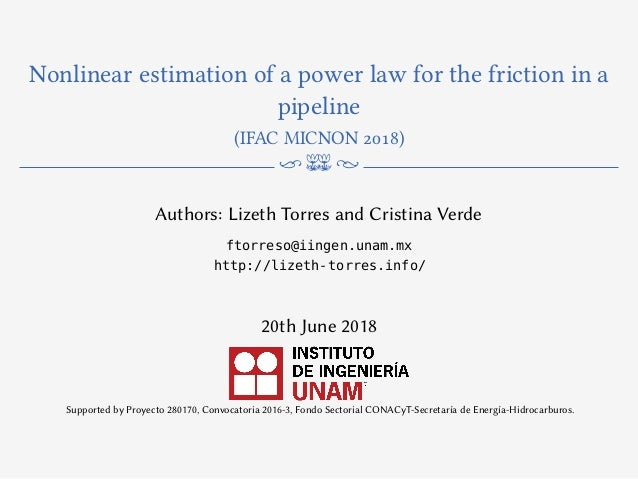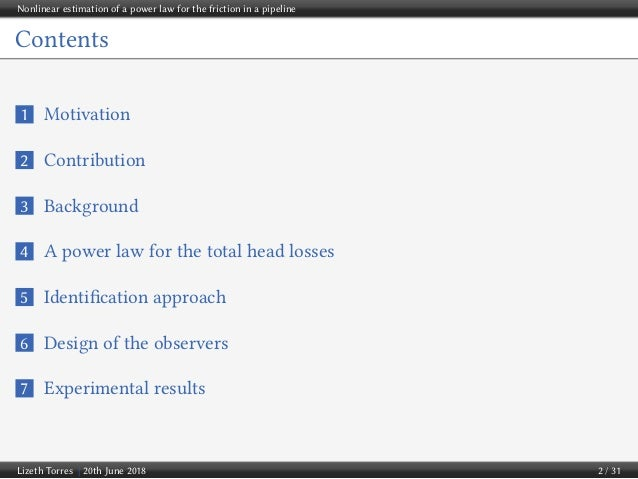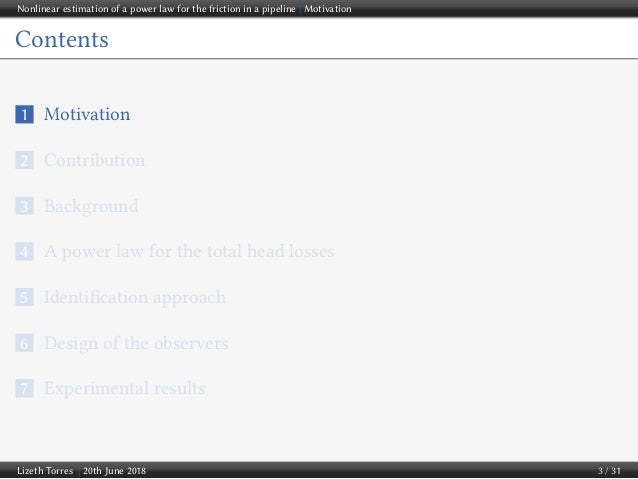Successfully reported this slideshow.Upcoming SlideShare
×

ofUpcoming SlideShare
Next

1 Like

Share

# Nonlinear estimation of a power law for the friction in a pipeline

See all

See all

### Nonlinear estimation of a power law for the friction in a pipeline

1. 1. Nonlinear estimation of a power law for the friction in a pipeline (IFAC MICNON 2018) n m N Authors: Lizeth Torres and Cristina Verde ftorreso@iingen.unam.mx http://lizeth-torres.info/ 20th June 2018 Supported by Proyecto 280170, Convocatoria 2016-3, Fondo Sectorial CONACyT-Secretaría de Energía-Hidrocarburos.
2. 2. Nonlinear estimation of a power law for the friction in a pipeline Contents 1 Motivation 2 Contribution 3 Background 4 A power law for the total head losses 5 Identification approach 6 Design of the observers 7 Experimental results Lizeth Torres | 20th June 2018 2 / 31
3. 3. Nonlinear estimation of a power law for the friction in a pipeline | Motivation Contents 1 Motivation 2 Contribution 3 Background 4 A power law for the total head losses 5 Identification approach 6 Design of the observers 7 Experimental results Lizeth Torres | 20th June 2018 3 / 31
4. 4. Nonlinear estimation of a power law for the friction in a pipeline | Motivation Motivation of the research Contributing to the proper management of distribution pipelines. A proper management requires some tasks such as ... The diagnosis and prognosis of the pipeline components. The control for the adequate distribution of the fluids. The solution of optimization problems. ⇓ Algorithms based on models formulated from physical laws that govern the flow behavior throughout the pipelines. Lizeth Torres | 20th June 2018 4 / 31
5. 5. Nonlinear estimation of a power law for the friction in a pipeline | Motivation Disadvantage Algorithms based on governing equations need to be frequently updated to avoid being invalid because of the nonstop use and the passing of time. Energy dissipation changes. Affected Parameters Roughness εa . Diameter φ. a It is not simple to measure it Aging deterioration consequences Corrosion Tuberculation Erosion Mineral deposits Lizeth Torres | 20th June 2018 5 / 31
6. 6. Nonlinear estimation of a power law for the friction in a pipeline | Motivation Motivation Main goal of this article Proposing an algorithm to continuously estimate the energy dissipation in a pipeline without measuring ε, φ and L. Why? To avoid miscalculations due to changes of the physical parameters that could affect the performance of model-based algorithms. Lizeth Torres | 20th June 2018 6 / 31
7. 7. Nonlinear estimation of a power law for the friction in a pipeline | Contribution Contents 1 Motivation 2 Contribution 3 Background 4 A power law for the total head losses 5 Identification approach 6 Design of the observers 7 Experimental results Lizeth Torres | 20th June 2018 7 / 31
8. 8. Nonlinear estimation of a power law for the friction in a pipeline | Contribution Contribution A power law to represent the energy dissipation mainly caused by friction in a pipeline. A simple method to estimate such a power law with the following characteristics: 1 It is based on nonlinear state observers. 2 It only requires two pressure head recordings and a flow rate measurement. 3 It performs the estimation in short-time. Lizeth Torres | 20th June 2018 8 / 31
9. 9. Nonlinear estimation of a power law for the friction in a pipeline | Background Contents 1 Motivation 2 Contribution 3 Background 4 A power law for the total head losses 5 Identification approach 6 Design of the observers 7 Experimental results Lizeth Torres | 20th June 2018 9 / 31
10. 10. Nonlinear estimation of a power law for the friction in a pipeline | Background The classic way to compute the energy dissipation In pipelines, energy dissipation is called head loss and can be divided into two main categories: major losses associated with energy loss per length of pipe and minor losses associated with bends, fittings, valves, that act against the fluid and reduce its energy. ∆Htot = ∆Hf + ∆Hm ∆Htot = f L φ v2 2g + N i=1 ki v2 2g = f L φ + N i=1 ki v2 2g ∆Htot =   f L + φ N i=1 ki f φ    v2 2g *Darcy-Weisbach equation Lizeth Torres | 20th June 2018 10 / 31
11. 11. Nonlinear estimation of a power law for the friction in a pipeline | Background Total head loss in pipeline If Leq = L + φ N i=1 ki f then ∆Htot = f Leq φ v2 2g Leq is known as equivalent length, which actually is the virtual length of a pipeline with devices as if it were a horizontal pipeline without devices. Note: If a device (e.g. a valve) in the pipeline is added or changed, the equivalent length will change and consequently the total head loss. The computation of f depends on the flow regime. Lizeth Torres | 20th June 2018 11 / 31
12. 12. Nonlinear estimation of a power law for the friction in a pipeline | Background Total head loss in pipeline For laminar flow: f = κ/Re where κ depends on the pipeline geometry. For circular pipes κ = 64. For turbulent flow (natural regime in pipelines): f can be accurately calculated by using the Colebrook-White (CW) equation 1 f = −2 log10 ε 3.71φ + 2.51 Re f Some disadvantages: ε is required, it is an implicit equation, it represents the complete turbulent regimen of the Moody diagram! We don’t need that, we only need to represent ∆Htot for the region where our pipelines work. These disadvantages are reasons to propose new formulas to represent ∆Htot. Lizeth Torres | 20th June 2018 12 / 31
13. 13. Nonlinear estimation of a power law for the friction in a pipeline | Background Another reasons to propose new formulas for ∆Htot An identifiable equation To have an equation with empirical parameters that are easy to estimate A differentiable equation Some optimization and identification algorithms require the derivative of the friction. Lizeth Torres | 20th June 2018 13 / 31
14. 14. Nonlinear estimation of a power law for the friction in a pipeline | Background Some proposed alternatives 1 Hazen William equation ∆Htot = 10.67 C1.852 LeqQ1.852 φ4.8704 2 Wood equation ∆Htot = (a(ε) + b(ε)Re−c(ε) ) f Q2Leq 2gφA2 r 3 Valiantzas equation ∆Htot = Leq k0Q2 φ5.3 m , k0 = 0.0126ε0.3 4 Quadratic (Prony) equation ∆Htot = aQ2 + bQ 5 Rojas equations 1 ∆Htot = Leq 2gφA2 r (θ1Q2 |Q| + θ2Q|Q|), ∆Htot = Leq 2gφA2 r θ1Q|Q| + θ2|Q| 1 Rojas Jorge et al. On-Line Head Loss Identification for Monitoring of Pipelines, Safeprocess 2018, Poland. Lizeth Torres | 20th June 2018 14 / 31
15. 15. Nonlinear estimation of a power law for the friction in a pipeline | A power law for the total head losses Contents 1 Motivation 2 Contribution 3 Background 4 A power law for the total head losses 5 Identification approach 6 Design of the observers 7 Experimental results Lizeth Torres | 20th June 2018 15 / 31
16. 16. Nonlinear estimation of a power law for the friction in a pipeline | A power law for the total head losses Our proposition ∆Htot = ΩQ1+γ, where Ω = α β . α, β and γ are parameters to be estimated, which can be related to the pipeline and fluid characteristics by using other equations for head losses. For the DW equation: γ = 1, α = f (Q) 2φArQγ−1 , β = gAr Leq . For the HW equation: Ω = 10.67Leq C1.852φ4.8704 , γ = 0.852. A general formula for friction losses It involves other head loss equation. Lizeth Torres | 20th June 2018 16 / 31
17. 17. Nonlinear estimation of a power law for the friction in a pipeline | Identification approach Contents 1 Motivation 2 Contribution 3 Background 4 A power law for the total head losses 5 Identification approach 6 Design of the observers 7 Experimental results Lizeth Torres | 20th June 2018 17 / 31
18. 18. Nonlinear estimation of a power law for the friction in a pipeline | Identification approach Approach description The least squares approach: Steps To obtain recordings (Time Series Data) of Q and ∆Htot at different operation points (from the lowest to the highest points). To obtain the mean of each recording: ¯Q, ¯∆Htot. To fit ¯Q vs ∆Htot by using least squares for instance. 0 200 400 600 800 1000 1200 1400 0 5 10 15 20 [m] Htot 0 200 400 600 800 1000 1200 1400 [s] 2 4 6 8 10 12 [m3 /s] 10 -3 Q 2 3 4 5 6 7 8 9 10 11 12 Q [L] 0 2 4 6 8 10 12 14 16 18 20 Htot [m] Experimental Data MATLAB fitted curve Lizeth Torres | 20th June 2018 18 / 31
19. 19. Nonlinear estimation of a power law for the friction in a pipeline | Identification approach Approach description The proposed approach: Steps To induce steady-oscillatory flow in the pipeline provoking a sinusoidal pressure at the upstream end. To estimate β and γ by using a nonlinear observer. To estimate α by using an algebraic equation or another nonlinear observer. ∆Htot = ΩQ1+γ, where Ω = α β . Advantages 1 The time required for the estimation is shorter. 2 It is not necessary to set the pipeline at lowest and highest operation points. Lizeth Torres | 20th June 2018 19 / 31
20. 20. Nonlinear estimation of a power law for the friction in a pipeline | Identification approach OBSERVER 1 Mean OBSERVER 2 Mean totH Q Q Q totH Q       tot in outH H H   inH outH Q d dt Lizeth Torres | 20th June 2018 20 / 31
21. 21. Nonlinear estimation of a power law for the friction in a pipeline | Design of the observers Contents 1 Motivation 2 Contribution 3 Background 4 A power law for the total head losses 5 Identification approach 6 Design of the observers 7 Experimental results Lizeth Torres | 20th June 2018 21 / 31
22. 22. Nonlinear estimation of a power law for the friction in a pipeline | Design of the observers Design of the observers Momentum equation: ˙Q = gAr Leq ∆Htot − Js(Q) Js(Q) is the dissipation term that depends on the head losses formula used (e.g. Js = f (Q)/2φArif DW equation is used). By substituting our proposed power law in the momentum equation ˙Q = β∆Htot − αQ|Q|γ If only positive flow is considered, then ˙Q = β∆Htot − αQ1+γ Lizeth Torres | 20th June 2018 22 / 31
23. 23. Nonlinear estimation of a power law for the friction in a pipeline | Design of the observers 1st Observer: β and γ estimation ˙Q = β∆Htot − αQ1+γ By x1 = Q, x2 = β, x3 = αQγ, x4 = γ, we get ˙x(t)=     0 u1 −y 0 0 0 0 0 0 0 0 0 0 0 0 0     x +     0 0 x3x4u2/y 0     y = 1 0 0 0 x (1) where u1 = ∆Htot and u2 = ˙Q is the derivative of the flow rate measurement. ˙x(t) = A(u, y)x + B(u, x) y = Cx Lizeth Torres | 20th June 2018 23 / 31
24. 24. Nonlinear estimation of a power law for the friction in a pipeline | Design of the observers It has already been proven by Torres et al. 20122 that under excitation condition, with u bounded and making A(u, y) bounded, if B(u, x) is globally Lipschitz in z uniformly in u, one can obtain an estimation of the state for system with the high-gain Kalman-like observer given by ˙ˆx = A(u, y)ˆx + B(u, ˆx) − Λ(λ)SCT (u)(ˆy − y) ˆy = Cˆx ˙S = λ(θS + [A(u, y) + dBλ(u, ˆx)]S + S[A(u, y) + dBλ(u, ˆx)]T − SCT CS), with Λ(λ) =      λIN1 0 λ2 IN2 ... 0 λq INq      , dBλ = 1 λ Λ−1 (λ) ∂B ∂x Λ(λ), ˆx(0) ∈ RN , S(0) ≥ 0, which ensure x(t) − ˆx(t) ≤ µe−σt , µ > 0, ∀t ≥ 1 λ . 2 Torres, L., Besançon, G. and Georges, D. (2012). EKF-like observer with stability for a class of nonlinear systems. IEEE Transactions on Automatic Control, 57(6), 1570-1574. Lizeth Torres | 20th June 2018 24 / 31
25. 25. Nonlinear estimation of a power law for the friction in a pipeline | Design of the observers 2nd Observer: α estimation ˙Q = β∆Htot − αQ1+γ By defining x1 = Q, x2 = α, we get system ˙x = 0 yˆγ|y| 0 0 x + ˆβu1 0 , y = 1 0 x(t) = Q, (2) where ˆβ and ˆγ represent the parameters estimated in the previous step. u1 = ∆Htot. To estimate the states of such a system, we used an exponential proposed by Besançon19963: 3 Besançon, G., Bornard, G., and Hammouri, H. (1996). Observer synthesis for a class of nonlinear control systems. European Journal of control, 2(3), 176-192. Lizeth Torres | 20th June 2018 25 / 31
26. 26. Nonlinear estimation of a power law for the friction in a pipeline | Experimental results Contents 1 Motivation 2 Contribution 3 Background 4 A power law for the total head losses 5 Identification approach 6 Design of the observers 7 Experimental results Lizeth Torres | 20th June 2018 26 / 31
27. 27. Nonlinear estimation of a power law for the friction in a pipeline | Experimental results Laboratory Pipeline Installations @ II-UNAM Physical parameters φ = 0.076 [m], L = 163.62 [m]. Lizeth Torres | 20th June 2018 27 / 31
28. 28. Nonlinear estimation of a power law for the friction in a pipeline | Experimental results 0 50 100 150 200 250 300 350 400 450 5 10 15 20 [m] Δ Htot 0 50 100 150 200 250 300 350 400 450 [s] 6 8 10 12 [m3 /s] ×10-3 Q Lizeth Torres | 20th June 2018 28 / 31
29. 29. Nonlinear estimation of a power law for the friction in a pipeline | Experimental results 0 50 100 150 200 250 300 350 400 450 [s] -2 -1 0 1 2 3 4 ×10-4 β 0 50 100 150 200 250 300 350 400 450 [s] 0.05 0.1 0.15 0.2 0.25 0.3 0.35 0.4 0.45 0.5 γ 0 50 100 150 200 250 300 350 400 450 [s] 0 0.5 1 1.5 2 2.5 3 α Parameter average ¯β = 2.2941 × 10−4, ¯γ = 0.4403, ¯α = 2.5599. Identified Momentum Equation ˙Q = 2.56∆Htot − 0.4403Q1.44 Lizeth Torres | 20th June 2018 29 / 31
30. 30. Nonlinear estimation of a power law for the friction in a pipeline | Experimental results Experimental Results 2 4 6 8 10 12 Q [L] 0 2 4 6 8 10 12 14 16 18 20 ΔH tot Experimental Data Power Law MATLAB fitted curve 3 4 5 6 7 8 9 10 11 12 Q [L] ×10-3 2 4 6 8 10 12 14 16 18 ΔH tot Steady State Points Steady Oscillatory Flow Lizeth Torres | 20th June 2018 30 / 31
31. 31. Nonlinear estimation of a power law for the friction in a pipeline | Experimental results qatlho’ Danke谢谢 Grazie Спасибо ขอบคุณ 9C4#5\$Ì ‫ﺷﻜﺮا‬ Merçi Gracias நன்றி Obrigado Ευχαριστώ 감사합니다 ध यवाद Terima kasih Thank you ありがとう Tapadh leibh ཐུགས་རྗེ་ཆེ་། Go raibh maith agaibh Xin cảm ơn Questions? ftorreso@iingen.unam.mx http://lizeth-torres.info Lizeth Torres | 20th June 2018 31 / 31
•Aug. 2, 2018

Total views

353

On Slideshare

0

From embeds

0

Number of embeds

285

2

Shares

0